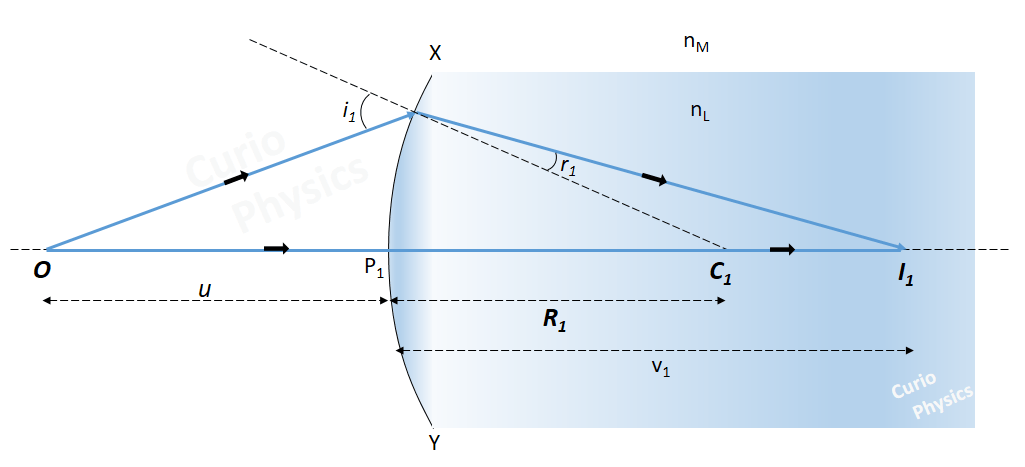# Lens makers formula

##### Lens maker’s formula

Lens makers formula :- Lens maker’s formula is the relation between the focal length of a lens to the refractive index of its material and the radii of curvature of its two surfaces. It is used by lens manufacturers to make the lenses of particular power from the glass of a given refractive index.

Let us suppose that a double convex lens made of glass of refractive index nL is placed in a rarer medium of refractive index nM. Below figure shows the geometry of image formation by the lens.The image formation can be seen in terms of two steps :-
(i) The first refracting surface forms the image I1 of the object O

If the lens material were continuous and there were no boundary/second surface XP2Y of the lens, then the refraction would take place only at surface  XP1Y and the refracted ray would go straight meeting the ray going along principle axis at point I1. Therefore I1 would have been a real image of O formed after refraction at XP1Y as shown in figure below :-Now using the formula of refraction at spherical surface, i.e.,$\displaystyle \frac{{{n}_{\text{material of refracted ray}}}}{v}-\frac{{{n}_{\text{material of incident ray}}}}{u}=\frac{{{n}_{\text{material of refracted ray}}}-{{n}_{\text{material of incident ray}}}}{R}$

Here nmaterial of refracted ray = nL and nmaterial of incident ray = nM

Object distance P1O = u and image distance P1I1 = v1

So we get$\displaystyle \frac{{{n}_{L}}}{{{v}_{1}}}-\frac{{{n}_{M}}}{u}=\frac{{{n}_{L}}-{{n}_{\text{M}}}}{{{R}_{1}}}$     …..(1)

But actually the lens material is not continuous. Therefore the refracted ray again suffers refraction at surface XP2Y of the lens, meeting actually the principle axis at I. Therefore I is the final real image of O, formed after refraction through the convex lens as shown in figure below :-For refraction at the second surface XP2Y, we can consider I1 as a virtual object, whose real image is formed at I.

Again using$\displaystyle \frac{{{n}_{\text{material of refracted ray}}}}{v}-\frac{{{n}_{\text{material of incident ray}}}}{u}=\frac{{{n}_{\text{material of refracted ray}}}-{{n}_{\text{material of incident ray}}}}{R}$

Now nmaterial of refracted ray = nM and nmaterial of incident ray = nL

Object distance P2I1 ≅ P1I1 = v1 and image distance P2I = v

So we get$\displaystyle \frac{{{n}_{M}}}{v}-\frac{{{n}_{L}}}{{{v}_{1}}}=\frac{{{n}_{M}}-{{n}_{\text{L}}}}{{{R}_{2}}}$     …..(2)

Adding equation (1) and (2), we get$\displaystyle \left[ \frac{{{n}_{L}}}{{{v}_{1}}}-\frac{{{n}_{M}}}{u} \right]+\left[ \frac{{{n}_{M}}}{v}-\frac{{{n}_{L}}}{{{v}_{1}}} \right]=\left[ \frac{{{n}_{L}}-{{n}_{\text{M}}}}{{{R}_{1}}} \right]+\left[ \frac{{{n}_{M}}-{{n}_{\text{L}}}}{{{R}_{2}}} \right]$$\displaystyle \Rightarrow \frac{{{n}_{M}}}{v}-\frac{{{n}_{M}}}{u}=\left[ \frac{{{n}_{L}}-{{n}_{\text{M}}}}{{{R}_{1}}} \right]-\left[ \frac{{{n}_{L}}-{{n}_{\text{M}}}}{{{R}_{2}}} \right]$$\displaystyle {{n}_{M}}\left( \frac{1}{v}-\frac{1}{u} \right)=\left( {{n}_{L}}-{{n}_{\text{M}}} \right)\left[ \frac{1}{{{R}_{1}}}-\frac{1}{{{R}_{2}}} \right]$$\displaystyle \Rightarrow \left( \frac{1}{v}-\frac{1}{u} \right)=\left( \frac{{{n}_{L}}}{{{n}_{M}}}-1 \right)\left[ \frac{1}{{{R}_{1}}}-\frac{1}{{{R}_{2}}} \right]$

Now put nL/nM = n = refractive index of material of the lens w.r.t. surrounding medium.

So$\displaystyle \Rightarrow \left( \frac{1}{v}-\frac{1}{u} \right)=\left( n-1 \right)\left[ \frac{1}{{{R}_{1}}}-\frac{1}{{{R}_{2}}} \right]$     …..(3)

When object on the left of lens is at infinity, image is formed at the principle focus of the lens.

∴ when u = ∞,  v = f = focal length of the lens.

From equation (3),$\displaystyle \left( \frac{1}{f}-\frac{1}{\infty } \right)=\left( n-1 \right)\left[ \frac{1}{{{R}_{1}}}-\frac{1}{{{R}_{2}}} \right]$

Or$\displaystyle \frac{1}{f}=\left( n-1 \right)\left[ \frac{1}{{{R}_{1}}}-\frac{1}{{{R}_{2}}} \right]$

This is the Lens Makers Formula.